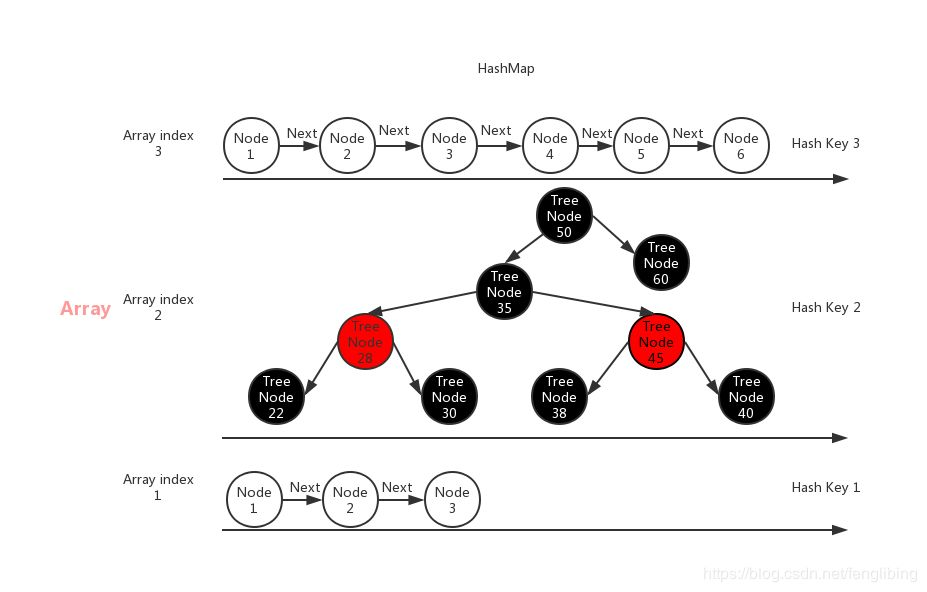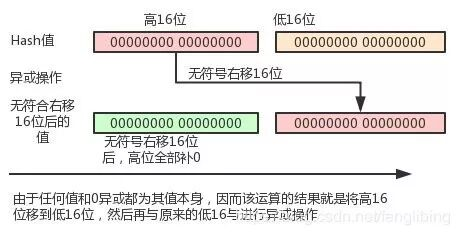HashMap 实现原理解读

（给ImportNew加星标，提高Java技能）

blog.csdn.net/fenglibing/article/details/91565912

HashMap是Java开发当中使用得非常多的一种数据结构，因为其可以快速的定位到需要查找到数据，其最快的速度可以达到O(1)，最差的时候也可以达到O(n)。本文以Java8中的HashMap做为分析原型，因为不同的JDK版本中的HashMap，可能存在着底层实现上的不一样。

HashMap是通过数组存储所有的数据，每个元素所存放数组的下标，是根据该存储元素的key的Hash值与该数组的长度减去1做与运算，如下所示：

index = (length_of_array - 1) & hash_of_the_key;

Node：

static class Node<K,V> implements Map.Entry<K,V> {
final int hash;
final K key;
V value;
Node<K,V> next;

Node(int hash, K key, V value, Node<K,V> next) {
this.hash = hash;
this.key = key;
this.value = value;
this.next = next;
}
......
}

hash值：当前Node的Hash值
key：当前Node的key
value:当前Node的value
next:表示指向下一个Node的指针，相同hash值的Node，通过next进行遍历查找

TreeNode：

static final class TreeNode<K,V> extends LinkedHashMap.Entry<K,V> {
TreeNode<K,V> parent;  // red-black tree links
TreeNode<K,V> left;
TreeNode<K,V> right;
TreeNode<K,V> prev;    // needed to unlink next upon deletion
boolean red;
TreeNode(int hash, K key, V val, Node<K,V> next) {
super(hash, key, val, next);
}
......
}//写入数据
public V put(K key, V value) {
//首先根据hash方法，获取对应key的hash值，计算方法见后面
return putVal(hash(key), key, value, false, true);
}

final V putVal(int hash, K key, V value, boolean onlyIfAbsent,boolean evict) {
Node<K,V>[] tab; Node<K,V> p; int n, i;
//判断用户存放元素的数组是否为空
if ((tab = table) == null || (n = tab.length) == 0)
//为空则进行初使化，并将初使化后的数组赋值给变量tab，数组的长值赋值给变量n
n = (tab = resize()).length;
//判断根据hash值与数组长度减1求与得到的下标，
//从数组中获取元素并将其赋值给变量p(后续该变量p可以继续使用)，并判断该元素是否存在
if ((p = tab[i = (n - 1) & hash]) == null)
//如果不存在则创建一个新的节点，并将其放到数组对应的下标中
tab[i] = newNode(hash, key, value, null);
else {//根据数组的下标取到了元素，并且该元素p且不为空，下面要判断p元素的类型是Node还是TreeNode
Node<K,V> e; K k;
//判断该数组对应下标取到的第一值是不是与正在存入值的hash值相同、
//key相等（可能是对象，也可能是字符串），如果相等，则将取第一个值赋值给变量e
if (p.hash == hash &&
((k = p.key) == key || (key != null && key.equals(k))))
e = p;
//判断取的对象是不是TreeNode，如果是则执行TreeNode的put方法
else if (p instanceof TreeNode)
e = ((TreeNode<K,V>)p).putTreeVal(this, tab, hash, key, value);
else {//是普通的Node节点，
//根据next属性对元素p执行单向链表的遍历
for (int binCount = 0; ; ++binCount) {
//如果被遍历的元素最后的next为空，表示后面没有节点了，则将新节点与当前节点的next属性建立关系
if ((e = p.next) == null) {
//做为当前节点的后面的一个节点
p.next = newNode(hash, key, value, null);
//判断当前节点的单向链接的数量（8个）是不是已经达到了需要将其转换为TreeNode了
if (binCount >= TREEIFY_THRESHOLD - 1) // -1 for 1st
//如果是则将当前数组下标对应的元素转换为TreeNode
treeifyBin(tab, hash);
break;
}
//判断待插入的元素的hash值与key是否与单向链表中的某个元素的hash值与key是相同的，如果是则退出
if (e.hash == hash &&
((k = e.key) == key || (key != null && key.equals(k))))
break;
p = e;
}
}
//判断是否找到了与待插入元素的hash值与key值都相同的元素
if (e != null) { // existing mapping for key
V oldValue = e.value;
//判断是否要将旧值替换为新值
if (!onlyIfAbsent || oldValue == null)
//满足于未指定不替换或旧值为空的情况，执行将旧值替换为新值
e.value = value;
afterNodeAccess(e);
return oldValue;
}
}
++modCount;
if (++size > threshold)
resize();
afterNodeInsertion(evict);
return null;
}

Hash值的计算方法：

// 计算指定key的hash值，原理是将key的hash code与hash code无符号向右移16位的值，执行异或运算。
// 在Java中整型为4个字节32位，无符号向右移16位，表示将高16位移到低16位上，然后再执行异或运行，也
// 就是将hash code的高16位与低16位进行异或运行。
// 小于等于65535的数，其高16位全部都为0，因而将小于等于65535的值向右无符号移16位，则该数就变成了
// 32位都是0，由于任何数与0进行异或都等于本身，因而hash code小于等于65535的key，其得到的hash值
// 就等于其本身的hash code。
static final int hash(Object key) {
int h;
return (key == null) ? 0 : (h = key.hashCode()) ^ (h >>> 16);
}** **

public V get(Object key) {
Node<K,V> e;
//根据Key获取元素
if ((e = getNode(hash(key), key)) == null)
return null;
if (accessOrder)
afterNodeAccess(e);
return e.value;
}

final Node<K,V> getNode(int hash, Object key) {
Node<K,V>[] tab; Node<K,V> first, e; int n; K k;
//if语句的第一个判断条件
if ((tab = table) != null //将数组赋值给变量tab，将判断是否为null
&& (n = tab.length) > 0 //将数组的长值赋值给变量n
&& (first = tab[(n - 1) & hash]) != null) {//判断根据hash和数组长度减1的与运算，计算出来的的数组下标的第一个元素是不是为空
//判断第一个元素是否要找的元素，大部份情况下只要hash值太集中，或者元素不是很多，第一个元素往往都是需要的最终元素
if (first.hash == hash && // always check first node
((k = first.key) == key || (key != null && key.equals(k))))
//第一个元素就是要找的元素，因为hash值和key都相等，直接返回
return first;
if ((e = first.next) != null) {//如果第一元素不是要找到的元，则判断其next指向是否还有元素
//有元素，判断其是否是TreeNode
if (first instanceof TreeNode)
//是TreeNode则根据TreeNode的方式获取数据
return ((TreeNode<K,V>)first).getTreeNode(hash, key);
do {//是Node单向链表，则通过next循环匹配，找到就退出，否则直到匹配完最后一个元素才退出
if (e.hash == hash &&
((k = e.key) == key || (key != null && key.equals(k))))
return e;
} while ((e = e.next) != null);
}
}
//没有找到则返回null
return null;
}

（点击标题可跳转阅读）

Java HashMap 遍历方式性能探讨

HashMap 和 HashTable 到底哪不同 ？

JDK7 与 JDK8 中 HashMap 的实现